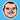Joe Abell

# Monoids

March 20, 2019

This post is a summary of what I have learned from https://marmelab.com/blog/2018/04/18/functional-programming-2-monoid.html. I have been been making a concerted effort in using this in my programming style recently, and it is making a difference in how my logic flows from one to another.

## Definition

• A monoid is a function that takes two values of the same type, and returns a third value of the same type. Numerical addition and string concatenation are examples.
• You should be able to break up a chain of monoids, run them concurrently, and as long as you run them in the same order, you should get the same result. (1 + 2) + 3 === 1 + (2 + 3).
• There should be a value that acts as a neutral value. An example of this is zero in addition. If you add zero to a twelve, you get twelve back. In string concatenation that is an empty string, in number multiplication the neutral value is 1. For a function, if the unit takes one value, the function is `a => a`.

## Benefits of Monoids

### Functional Composition

Other than the simple monoids mentioned above, we can make a monoid out of function composition. The compose method is one:

``const compose = (f1, f2) => arg => f1(f2(arg));``

Athough for readabily, I prefer to reverse the order the functions are run in a flow or composeRight function.

``const flow = (f1, f2) => arg => f2(f1(arg));``

If you use flow, your `f1` function will be run before `f2`. Let’s break down how flow meets the definition.

• If `f1` and `f2` are functions, flow returns a function.
• We can break up a chain of flows. Example incoming.
``````const flow = (f1, f2) => arg => f2(f1(arg));

const add1 = a => a + 1;
const double = a => a * 2;
const showResult = a => console.log(a);

• We have a neutral value: `val => val;`

## Monoids and Array.reduce are friends

We can use flow as our function to pass into Javascript’s reduce method. All we need is the neutral value to pass as the second argument to reduce. An examply here is using a multiply monoid with the neutral number of 1 to create the factorial of the passed in number.

As an example, I tried to create a factorial function without using recursion. The hardest bit is creating the data we will work with, the rest is simple.

``````// We need an array which has every integer from a given number back to 1.
// Array(5) gives us an array with empty items, which has a length of 5.
// Array.from() takes an array and a factory function, and uses the function
// populate each item into a new array.
// + 1 stops us from multiplying by zero.

const buildArray = num => Array.from(Array(num), (_, index) => index + 1);

const multiply = (a, b) => a * b;
const factorial = num => buildArray(num).reduce(multiply, 1);

factorial(5); // 120``````

## Monoids are great for async composition too

It is trivial to create an async version of the flow method.

``````const asyncFlow = (f1, f2) => async arg => await f2(await f1(arg));
const getData = async id => await fetch(`http://icndb.com/jokes\${id}`);
const getJson = async response => await response.json();

const data = asyncFlow(getData, getJson)(23);``````